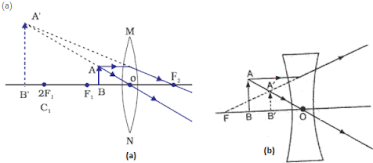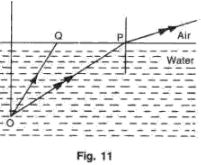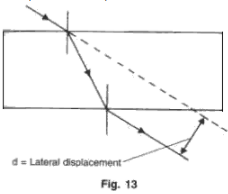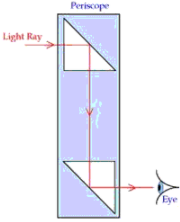# Frank Solutions and MCQ for Chapter 2 Light Class 10 Physics ICSE

## Exercises

1. Make the correct choices in the following items:

(a) A magnified erect image is obtained when an object is positioned in front of a converging lens. The distance of the object from the lens would be
(i) Greater than three focal lengths.
(ii) Equal to two focal lengths.
(iii) Between one and two focal lengths.
(iv) Equal to one focal length.
(v) Less than one focal length.
(v) less than one focal length

(b) An object is placed 50 cm from a converging lens of focal length 30 cm . The image produced would be
(i) Inverted and same size as the object .
(ii) Inverted and diminished.
(iii) Inverted and magnified.
(iv) Erect and diminished.
(v) Erect and magnified.
(iii) inverted and magnified.

(c) In fig. 1, a real image of a point object O is formed. Which of the following statements is true about each of the arrangements?(i) The object is at the principle focus of the lens.
(ii) The focal length of the lens is 17 cm.
(iii) If the mirror is moved so that it is 6 cm from the lens  image will also move.
(i), (ii) and (iii)

(d) A lens used as a magnifying glass
(i) Is a diverging lens.
(ii) Produces a virtual image.
(iii) Is placed with the object nearer the lens than the principle focus.
(ii) and (iii) only

(e) If the image can be focused on a screen it must be
(i) Erect
(ii) Virtual
(iii) Real
(iv) Upright
(v) Coloured
(ii) real

(f) The image formed by a diverging lens, shown in fig. 2, is
(ii) virtual, erect, diminished

(g) A converging lens B has the same focal length as a converging lens A, but only half the diameter. Both lens are used, separately, to form two images of building on a screen. Which one of the following statements about the images is correct ?
(i) The image B formed is closer to the lens than the image formed by A.
(ii) The image B formed is bigger than the image formed by A.
(iii) The image B formed is not as sharply focused as the image formed by A. /
(iv) The images are of the same brightness.
(v) Both the images are real and inverted.
(v) Both the images are real and inverted.

2. A pin 2 cm long is placed 12 cm away from a convex lens at right angles to the principal axis. If the focal length of the lens is 20 cm, by scale drawing find the size of the image and its magnification.
Given O = 2 cm , u = 12 cm, f = 20 cm
I = ?, M = ?
Taking the scale of measurement are : 2 cm = 1cm on the graph.
In the drawing,
O = 1 cm, u = 6 cm, f = 10 cm
From the drawing,
I = 2.7 cm
⇒ Actual image size I = 2.7 × 2 = 5.4 cm

3. (a) Define the term 'focus' of a lens.
(b) Distinguish between concave and convex lens.

(a) Principal focus: Rays of light can pass through the lens in any direction and hence there will be two principal foci on either side of the lens and they are referred to as the first principal focus and the second principal focus of a lens.
First Principal Focus (F1)
It is a point on the principal axis of the lens such that the rays of light starting from it (convex lens) or appearing to meet at the point (concave lens) after refraction from the two surfaces of the lens become parallel to the principal axis of the lens.
Second Principal Focus (F2)
It is a point on the principal axis of the lens such that the rays of light parallel to the principal axis of the lens after refraction from both the surfaces of the lens pass through this point (convex lens) or appear to be coming from this point.
(b)

 Convex lens Concave lens 1. A convex lens is thicker in the middle and thinner at its edges.2. A light beam converges on passing through a convex lens.3. The convex lens produces a real image that is inverted and is smaller and can be obtained on screen. 1. A concave lens is thicker at the edges and thinner in the middle.2. A light beam diverges on passing through a concave lens.3. A concave lens always forms a virtual image, the image is magnified and cannot be obtained on screen.

4. (a) Draw a sketch to show how a lens is able to produce an image of the sun on a paper screen.
(b)(i) Would you regard the rays from the sun as being divergent, parallel or convergent?
(ii) What is the name given to the point where such rays meet after they have passed through the lens?
(iii) How does the image of the sun sometimes burn a paper screen?(b)
(i) Rays from sun can be regarded as parallel rays.
(ii) The point is called 'Focus'.
(iii) A convex lens is used to focus the sun rays on a piece of paper to burn a piece of paper. A large amount of heat gets concentrated at a point and is sufficient to burn the piece of paper.

5. (a) What type of a lens can be used as a magnifying glass?
(b) Show by a ray diagram the formation of a real image by simple magnifying lens.
(a) A convex lens with short focal length can be used as a magnifying glass.
(b) A simple magnifying glass forma a virtual, erect and magnified image of a tiny object which is distinctly seen by the eye because the eye lens converges the rays to form a real image on the retina.Ray diagram for the formation of image by a magnifying glass.

7. (a) What is meant by the power of accommodation produced?
(b) How is accommodation produced?
(c) What type of lenses are used in spectacles worn by an old lady for knitting?
(a) The image of the objects at different distances from the eye is brought to focus on the retina by changing the focal length of the eye lens. This is called the power of accommodation of the eye.

(b) Accommodation is achieved with the help of ciliary muscles.
To focus the distant objects, the ciliary muscles are relaxed causing the eye lens to become thin and thus increasing the focal length of the eye lens.
To form the image of a near object on the retina, the ciliary muscles contract and thereby pull the ends of the choroid closer. Thus, the eye lens thickens to shorten its focal length and converges the rays to form the image.
In this manner, by changing the focal length of the eye lens, the image of the objects at different distances is brought to focus on the retina.

(c) A converging lens of suitable focal length is used in the spectacles worn by an old lady for knitting.
9. An object is placed in front of a converging lens and in front of a diverging lens as in fig. 5.
(a) Complete the ray diagram to obtain an image.
(b) Compare the nature of image formed by both the lenses in the above case.
(a)(b) In both the cases the image formed is virtual, upright and on the same side of the lens but the image formed by a convex lens is magnified and that formed by a concave lens is diminished.

11.
 Gamma Rays D C Visible light B A
(f) Name one use of each of the radiation.The above table shows different parts of the electromagnetic spectrum.
(a) Identify the parts of the spectrum marked as A, B, C and D.
(b) Which of the radiations A or B has the higher frequency?
(c) State two properties which are common to all parts of the electromagnetic spectrum.
(d) Name one source of each of the radiation of electromagnetic spectrum.
(e) Name one detector for each of the radiation.
(a) A, B, C and D are microwaves, infrared waves, ultraviolet light and x-rays respectively.
(b) Radiations B (microwaves) have a higher frequency.
(c) Common properties of e-m spectrum:
1. All electromagnetic waves travel with the same speed in vacuum (or air) which is equal to the speed of light i.e. 3 x 108 m/s.
2. These waves are unaffected by the electric and magnetic fields.
(d)
 Name of wave Source 1. Gamma rays2. X-rays3. Ultravoilet4. Visible light5. Infrared-waves6. Microwaves7. Radio waves 1. Cosmic rays.2. When highly energetic electrons are stopped by a heavy metal target of hight melting point (x-ray tube).3.Sunlight4. White hot bodies,5. Lamp with thoriated filament.6. Electronic devices such as klystron tube.7. Radio transmissions

(e)

 Name of wave Detector 1. Gamma rays2. X-rays3. Ultravoilet4. Visible light5. Infrared waves6. Microwaves7. Radio waves 1. Geiger tube2. Photographic film coated with zinc sulphide3.Photographic plate4. Eye photo – cells5. Thermopile6. Wave guide tubes7. Earphone

(f)

 Name of wave Use 1. Gamma rays2.X-rays3. Ultravoilet4. Visible light5. Infrared waves6. Microwaves7. Radio waves 1. Detecting flaws in metal casting.2.Diffraction to find crystal structure.3. Burglar alarms.4. Photography.5. Infra – red photography6. Microwave cooking7. Communication and navigation.

13. Make the correct choice for each of  the following :

(a) Which one of the following (Fig. 7) correctly shows the path of the ray through the glass block ?
The (v) diagram correctly shows the path of the ray through the glass block

(b) Total internal reflection takes place when
(i) Light is going from glass to air and ∠I < ∠C
(ii) Light is going from air to glass and ∠i < ∠C
(iii) Light is going from glass to air and ∠i > ∠C
(iv) Light is going from air to glass and ∠i > ∠C
(v) Light is going from glass to air and  ∠i = ∠ r
where  ∠i = angle of incidence,
∠ r = angle of refraction,
∠ C = critical angle
(iii) light is going from glass to air and ∠i > ∠C

(c) with reference to the fig. 8, the refractive index of the glass block is :

(d) Fig. 9 illustrates an experiment to determine the refractive index of glass. Which one of the following graphs would give a straight line through the origin  ?
(i) ∠i plotted against ∠r.
(ii) Sin i plotted against ∠r.
(iii) ∠i plotted against sin D.
(iv) 1/∠i plotted against 1/∠r.
(iv) sin i plotted against sin r

(e) Which one of the five diagrams (Fig. 10) represents the path of a ray of light through a periscope ?
(iii) The third diagram represents the path of the light through the periscope.

14. Fig. 11 shows two rays of light Op and OQ coming from an object at the bottom of a pond, incident on the water surface.(a) Mark on the diagram
(i) The angle of incidence of ray OP,
(ii) The angle of refraction of ray Op,
(iii) The position of image of the object as seen from above.
(iv) An approximate path of the ray OQ.
(b) Explain, why do the rays of light change directions on passing from water to air.
(c) A fish in water sees everything outside the water by rays of light entering its eye in a small cone of light. Draw a diagram and explain how does this happen.

(i) Angle of incidence (i) of ray OP is marked in the above diagram.
(ii) Angle of refraction (r) of ray OP is marked in the above diagram.
(iii) The position of image of the object (O') as seen from above is marked in the diagram.
(iv) An approximate path of ray OQ is shown in the above diagram.
(b) Water is a denser medium as compared to air; so on passing from water (denser) to air (rarer) the speed of light of light increases and it bends away from the normal.
(c) Refraction is the bending of light as it passes from a medium of one optical density into a medium of a different optical density, as from air to water or water into air. The amount of bending is dependent upon the incident angle of the light. In the diagram below, a light ray, "A" strikes the water at right angles and passes through the surface without bending. But as the incident angle decreases (becomes less than 90 degrees) the light bends more and more rays "B" and "C." Light striking the surface parallel to the surface, bends downward.

Since, light is coming into the water from all directions, refraction creates a cone of light with its base on the surface and its apex at the fish's eye. The base of the cone is a circular opening at the surface through which the fish sees the entire outside world. This opening is called the "Fish's Window". Only the light passing through the window enters the fish's eye. Notice line "D," It's a ray entering the water beyond the window; refraction bends it such that it cannot reach the fish's eye.

15. (a)A ray of light is incident at 45° on the face of
(i) A rectangular block of glass.
(ii) A 60° glass prism.
(b) Draw a sketch showing how the ray of monochromatic ray of light passes through glass in each case.
(c) With the aid of a diagram, explain how the face of a right angled prism may totally reflect incident on it.
(d) A thick plane mirror produces several faint images in addition to a prominent one. Draw a ray diagram showing how reflection and refraction produce all these images.
(e) Fig. 12 represents a stone S at the bottom of a pond of water. Using the two rays, as shown, complete the ray diagram to show where the image of the stone appears when viewed from E.(f) What is a ''mirage'? Explain with the help of a diagram.
(g) A man observes the bottom of a swimming pool of 3 m depth. If the refractive index of water is 1.3, what is the apparent depth of water?
(h) When a ray of light undergoes refraction through a glass slab and when it emerges it is displaced laterally (Fig. 13). What are the factors on which the lateral displacement depends?(i) Fig. 14 shows three rays of light OA, OB and OC passing from water to air, making angles 49
°, 41° and 35° with the horizontal surface respectively. Draw an approximate path of the emergent ray for each. (Critical angle of water is 49°.)
(a)  For the glass block,
Given i = 45
Using μ = sin i/sin r
Taking μ = 1.5 for a glass, we get r = 28°
The ray diagram of parallel glass slab will look like this :(b) For Triangular glass prism :
Given i = 45
Using μ = sin i/ sin r
Taking μ = 1.5 for a glass, we get r = 28°
The ray diagram of triangular glass prism will look like this :In the above diagram, light rays from the object AB are incident normally on a right angled glass prism and hence they pass undeviated . These rays fall on the other surface of the prism at 45°,  which is greater than the critical angle for the glass-air interface (42°). Here, no refraction takes places but the ray of light is totally reflected back in the glass and finally it emerges out through the third surface of the prism normally forming the image A'B' of the object AB.(f) Mirage is a naturally occurring optical phenomenon caused due to total internal reflection light wherein an image some distant object appears displaced from its true position; ofter observed in deserts and coal - tarred roads on hot summer days.
In the desert, the air is very hot. The air near the surface of the earth is hot and is less dense. Thus, air can be considered as layers of medium with higher density in the vertical upward direction. Rays of light from an object, say, a tree bend away from the normal as they pass from the denser layer tot he rarer layer. The angle of incidence increases for successive layer. At a certain point, when the angle of incidence becomes greater than the critical angle, the rays of light will undergo total internal reflection. The ray now starts traveling from rarer to denser medium. When the light reaches the eyes of a weary dese traveler, to him, the light will appear to emerge in a straight line in the backward direction .
Thus, creating an impression of water pool or oasis.
(i) Given the angles with the horizontal surface as :
OA making an angle of 49° with horizontal, hence iA = 90° - 49° = 41°
OB making an angle of 41° with horizontal, hence iB = 90° - 41° = 49°
OC making an angle of 35° with horizontal, hence ic = 90° - 35° = 55°
Given critical angle  = 49°.
So all incident angles in denser media, more than 49°, will undergo total internal reflection.(Ray OC)
i = critical angle will graze through the interface (Ray OB).
i = less than critical angle will emerge out into rare medium due to refraction (Ray OA).

16. Define critical angle and explain total internal reflection. What are the conditions necessary for total internal reflection?
Critical angle: The angle of incidence in the denser medium for which the angle of refraction in the rarer medium is 90o.
Total internal reflection: It is an optical phenomenon that occurs when a ray of light strikes the boundary of denser medium at an angle greater than the critical angle w.r.t. the normal of the surface; this ray is then totally reflected back into the denser medium.
The two necessary conditions for total internal reflection to take place are:
1. The light ray must proceed from denser to rarer medium.
2. Angle of incidence in denser medium should be greater than the critical angle for the pair of media in contact.

17. (a) A ray of monochromatic light enters glass PQRS as shown in the fig. 15. Complete the path of ray till it emerges from the glass. (Critical angle of glass is 42°).
At angle of incidence equal to critical angle, the ray grazes the interface.
(b) Diagram of prism periscope :(c) In case of reflection from plane mirror, all of light is not reflected. In case of total reflecting prism, all rays falling beyond critical angle will be totally reflected. The intensity of reflected light is equal to the intensity of incident light in case of total internal reflection but not in case of reflection from plane mirror.
In addition, the reflection through a thick mirror causes multiple image formation as well as lateral inversion of image.
Due to the above two reasons, the total reflecting prisms are preferred over the plane mirrors.

18. Fig. 16 shows a ray of white light that passes through a prism and produces a spectrum.
(a) Name the phenomenon that is taking place.
(b) What colour would you see at X and Y?
(c) What radiation would you detect above X and below Y?

(a) Dispersion
(b) Red colour at X and violet colour at Y.
(c) Above X, we would detect infra-red radiation and below Y, ultra-violet radiation.

19. Give two properties of ultraviolet radiations which differ from the visible light.
Two properties of ultraviolet radiation different from the visible light:
1. Ultraviolet radiation can pass through quartz, but they are absorbed by glass.
2. They are usually scattered by the dust particles present in the atmosphere.

20. Mention three properties of infra red radiations similar to the visible light. Give two such properties of infrared radiations which are not true for visible light.

Three properties of infrared radiations similar to the visible light:
1. They travel in straight lines as light does, with a speed equal to 3 x 108 m/s in vacuum.
2. They obey laws of reflection and refraction.
3. They are unaffected by electric and magnetic fields.
Two properties of infrared radiation different from the visible light:
1. They are absorbed by glass, but they are not absorbed by rock-salt.
2. They are detected by their heating property using a thermopile or a blackened bulb thermometer.

21. Two waves A and B have wavelength 0.01Å and 9000 Å respectively.
Name the two waves. Compare the speeds of these waves when they travel in vacuum.
In vacuum, both travel with the same speed. Hence, the ratio of their speeds is 1:1.

24. Fig. 20 shows part of the arrangement for obtaining pure spectrum.(b)  A pure spectrum is that spectrum in which the different colours are distinctly seen without any overlapping. Following conditions must be satisfied to get a pure spectrum.
1. The slit (placed in from of the source ) must be as narrow as possible. A wide slit is equivalent to a large number  of narrow slits placed side by side. Each narrow slit will give its own spectrum. So, there will be overlapping of different spectra.
2. The rays of light in the incident beam must be parallel to each other. This is achieved by using a convex lens. A convex lens should be so placed that the slit is at its focus. The convex lens used in this way is called collimating lens while the beam emerging out of this lens is called collimated beam.
If the incident beam is parallel, then in the refracted beam, all the rays of the same colour will be parallel and will be focused separately.
3. The prism must be placed in the minimum deviation position. When the prism is placed in the position of minimum deviation, all the rays are deviated by equal amounts. This ensures freedom from overlapping.
4. On emergence from the prism, all rays of one colour should form a parallel beam of their own. If a convex lens is suitably placed in the path of these rays, then each parallel beam will come to its own focus. In this way, a pure spectrum will be obtained.

25. What are ultraviolet radiations? How are they detected? State two properties and one use of ultraviolet radiations.
The electromagnetic radiations of wavelength from 100 Å to 4000 Å are called the ultraviolet radiations.
Detection: If the different radiations from the red part of the spectrum to the violet end and beyond it are made incident on the silver-chloride solution, it is observed that from the red end to the violet end, the solution remains almost unaffected. However just beyond the violet end, it first turns violet and finally it becomes dark brown (or black). Thus, there exist certain radiations beyond the violet end of the spectrum, which are chemically more active than the visible light. These radiations are called the ultraviolet radiations.
1. Ultraviolet radiation can pass through quartz, but they are absorbed by glass.
2. They are usually scattered by the dust particles present in the atmosphere. One use of ultraviolet radiation: In producing, vitamin D, in food of plants and animals.

26. Name three properties of ultraviolet radiations which are similar to visible light.
Three properties of ultraviolet radiations similar to the visible light:
1. They travel in straight lines as light does, with a speed equal to 3 x 108 m/s in vacuum.
2. They obey laws of reflection and refraction.
3. They are unaffected by electric and magnetic fields.

27. An electromagnetic wave has a frequency of 500 MHz and a wavelength of 60 cm. Calculate the velocity of the wave. Name the medium through which it is traveling.